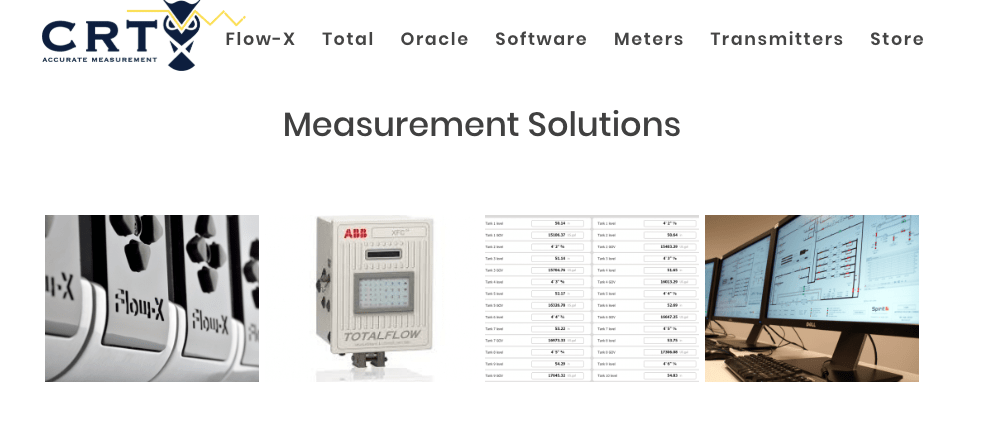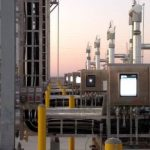## Volume Flow vs. Mass Flow

Volume flow is okay if your product is sold by volume. But mass flow measurement is simply the most accurate way of measuring the actual amount of a substance moving through a pipeline. Mass is related to volume by density. The greater the density of a substance, the larger its mass will be for the same volume. Mass equals volume times density. So simultaneous measurement of volume flow and density gives mass flow. Telling us exactly how much of a substance we have.

## High Viscosity Liquid Measurement

With a liquid like water, you can accurately access mass by measuring volume (mass flow measurement) because the density is known and doesn’t vary much. Many high viscosity liquids are sold by mass which is often mistakenly called weight, but that’s another story. Different grades of oil have different densities. By measuring the mass of a quantity of oil as it dispensed and dividing it by the volume, you can immediately verify the density and therefore the grade of oil you have bought. For that, you need to know the mass and a mass flowmeter will tell you this.

## Mass Flow: Quantitative and Qualitative Information

So in short, mass flow can tell you the quantitative and qualitative information about a liquid that volume flow cannot tell you. Now, where mass flow measurement from ABB really comes into its own is with gases. Although gases still have the same rules, mass equals volume times density. They simply refuse to behave. The volume of a pure gas under ideal conditions is directly proportional to its mass. So measuring the volume can tell you exactly the mass of the gas you have. Meanwhile, in the real world where gases are never pure and process conditions are never ideal, they’re all sorts of ways to calculate the amount of gas flowing in a pipeline from its volume.

##